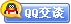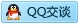##用户名 Email 自动登录 找回密码 密码 会员注册
 VIP会员，3年作业免费下 ！ 奥鹏作业，奥鹏毕业论文检测 新手作业下载教程，充值问题 没有找到答案，请在此处留言！ 2020年07月最新全国统考资料 投诉建议，加盟合作！奥鹏课程积分软件(ver:3.1)

# 11春高等数学备考试题库（免费奥鹏作业答案）发表于 2011-6-15 17:54:45 | 显示全部楼层 |阅读模式全国教师教育网络联盟入学联考 （专科起点升本科） 高等数学备考试题库 2011年 一、选择题 1. 设 的定义域为 ，则 的定义域为（     ）. A:   B:   C:   D:   2. 函数 的定义域为（      ）. A:   B:   C:   D:   3.下列说法正确的为（     ）. A: 单调数列必收敛； B: 有界数列必收敛； C: 收敛数列必单调； D: 收敛数列必有界. 4.函数 不是（    ）函数. A: 有界 B: 单调 C: 周期 D: 奇 5.函数 的复合过程为（    ）. A:   B:   C:   D:   6.设 ，则下面说法不正确的为(     ). A: 函数 在 有定义； B: 极限 存在； C: 函数 在 连续； D: 函数 在 间断。 7. 极限 = (      ). A: 1 B: 2 C: 3 D: 4 8. （     ）. A: 1 B: e C:   D:   9.函数 的图形对称于（     ）. A: ox轴； B: 直线y=x； C: 坐标原点; D: oy轴 10.函数 是（     ）. A: 奇函数； B: 偶函数； C: 有界函数； D: 周期函数. 11.下列函数中，表达式为基本初等函数的为（      ）. A:   B:   C:   D:   12.函数 是（       ）. A: 偶函数； B: 奇函数； C: 单调函数； D: 有界函数 13. （     ）. A: 1 B:   C:   D: 不存在 14.在给定的变化过程中，下列变量不为无穷大量是（     ）. A:   B:   C:   D:   15. （      ）. A: 1 B: e C:   D:   16.下面各组函数中表示同一个函数的是（     ）. A:  ; B:  ; C:   D:  ; 17.  （     ）. A: 1 B:   C:   D: 不存在 18.设 ，则下面说法正确的为(       ). A: 函数 在 有定义； B: 极限 存在； C: 函数 在 连续； D: 函数 在 可导. 19. 曲线   上点 (2, 3)处的切线斜率是（    ）. A: -2 B: -1 C: 1 D: 2 20. 已知 ，则 （     ）. A: -4 B: 4 C: 0 D: 1 21. 若 则  (      ). A: -1 B: 1 C: 2 D: -2 22. 函数 =  在定义区间内是严格单调（     ）. A: 增加且凹的 B: 增加且凸的 C: 减少且凹的 D: 减少且凸的 23.  在点 可导是 在点 可微的（     ）条件. A: 充分 B: 必要 C: 充分必要 D: 以上都不对 24. 上限积分 是（     ）. A:  的一个原函数 B:  的全体原函数 C:  的一个原函数 D:  的全体原函数 25.设函数 ，则 （     ）. A:  ; B: -1 C:   D:   26.  的导数  (       ). A:   B:   C:   D:   27. 已知  ，则 (      ). A: 2 B:   C:   D:   28. 设函数 在区间 上连续，则  （      ）. A:   B:   C:   D: 不能确定 29.  （      ）. A:   B:   C:   D:   30. 设 ，则偏导数 （     ）. A:   B:   C:   D:   31. 极限 =（      ）. A: 1 B: 2 C: 0 D: 3 32. 设函数 ，则  （  ）。 A:   B:   C:   D:   33. 曲线 的凸区间是（      ） A:   B:   C:   D:   34.  （      ） A:   B:   C:   D:   35.  （      ）. A:   B:   C:   D:   36 .上限积分 是（      ）. A:  的一个原函数 B:  的全体原函数 C:  的一个原函数 D:  的全体原函数 37. 设 的定义域是（      ）. A:   B:   C:   D:   38. 已知 ，则 （     ）. A: dx B: 2dx C: 3dx D:  dx 39. 函数 ，则 （      ）. A:   B:   C:   D: 以上都不对 40.  （      ）. A: 1 B: 4 C: 0 D: 2 41. 已知 ，则 （     ） A:   B:   C:   D:   42. 若函数 ，则 （    ）. A:   B:   C:   D:   43.  （     ）. A: 0 B: e C: 1 D: -e 44.  （     ）. A:   B:   C:   D:   45. 设 ，则偏导数 （     ）. A:   B:   C:   D:   二、填空题 1.                 . 2.                . 3. 函数 的反函数为              . 4.                 . 5.                 . 6.                . 7.                     . 8. 函数 的反函数为                . 9. 设   ， ,  则                . 10. 设 ， 则              . 11.                 . 12. 曲线 在点 处的切线方程是             . 13. 由方程 所确定的函数 在点 的导数是            . 14. 函数 的拐点是                . 15.  　　    　    　. 16.   　　    　    　. 17. 函数 的定义域为                . 18. 设 ，则                 . 19. 函数 的单调递减区间为___________ . 20. 函数 的驻点为          . 21. 函数 的单调增加区间是　　          　　 .   22. 设函数 在点 处具有导数，且在 处取得极值，则            . 23.                  . 24.                  . 25.                  . 26. 曲线 在点 处的切线方程是             . 27. 设由方程 可确定 是 的隐函数，则               . 28.                . 29.                . 30.函数 的定义域为                  . 31. 函数  的极大值是           . 32. 函数 的单调递增区间为                  . 33.               . 34.              . 35. 设 , 则             . 三、简答题 1. 计算  . 2. 求函数 的极值 3. 设 是连续函数，求 4.求 5.  设二元函数为 ，求 . 6.   计算  . 7. 已知 ，求 8. 设 且 存在，求 9. 求 。 10. 求   11. 计算  . 12.求函数  的极值 13.求 . 14. 求 . 15. 求 16. 求证函数  在点 处连续. 17. 设 ，求 的不连续点. 18. 设 ，若 存在，求 19. 设二元函数为 ，求 . 久爱奥鹏网：www.92open.com
 本版积分规则 回帖并转播 回帖后跳转到最后一页客服一客服二客服三客服四微信客服扫一扫